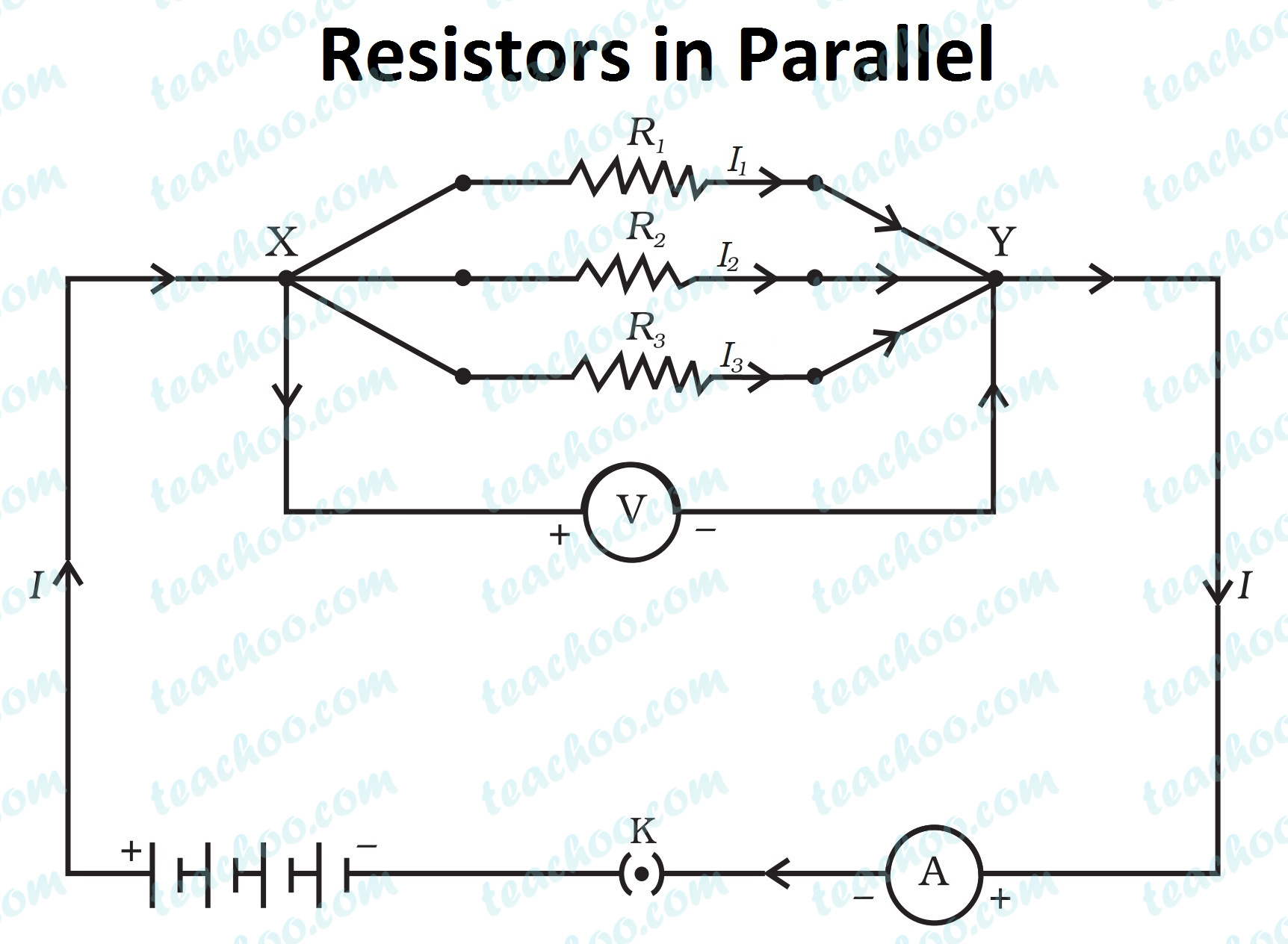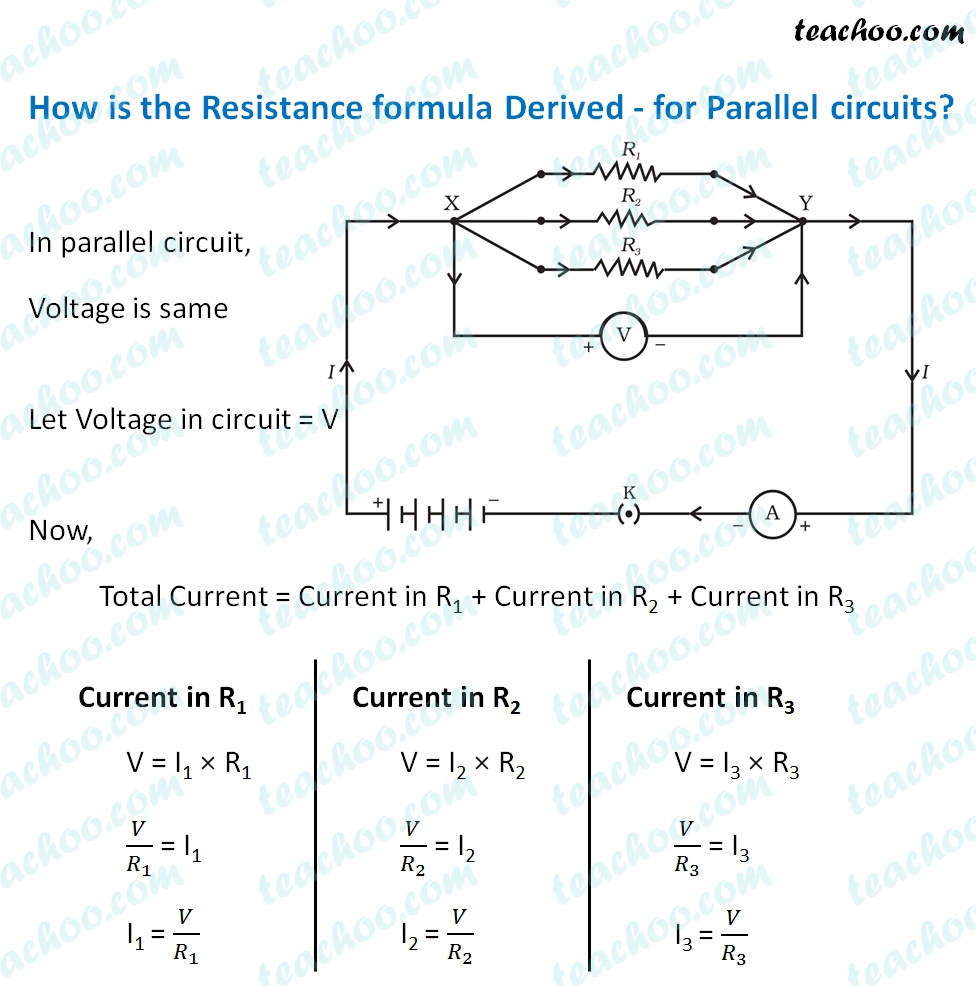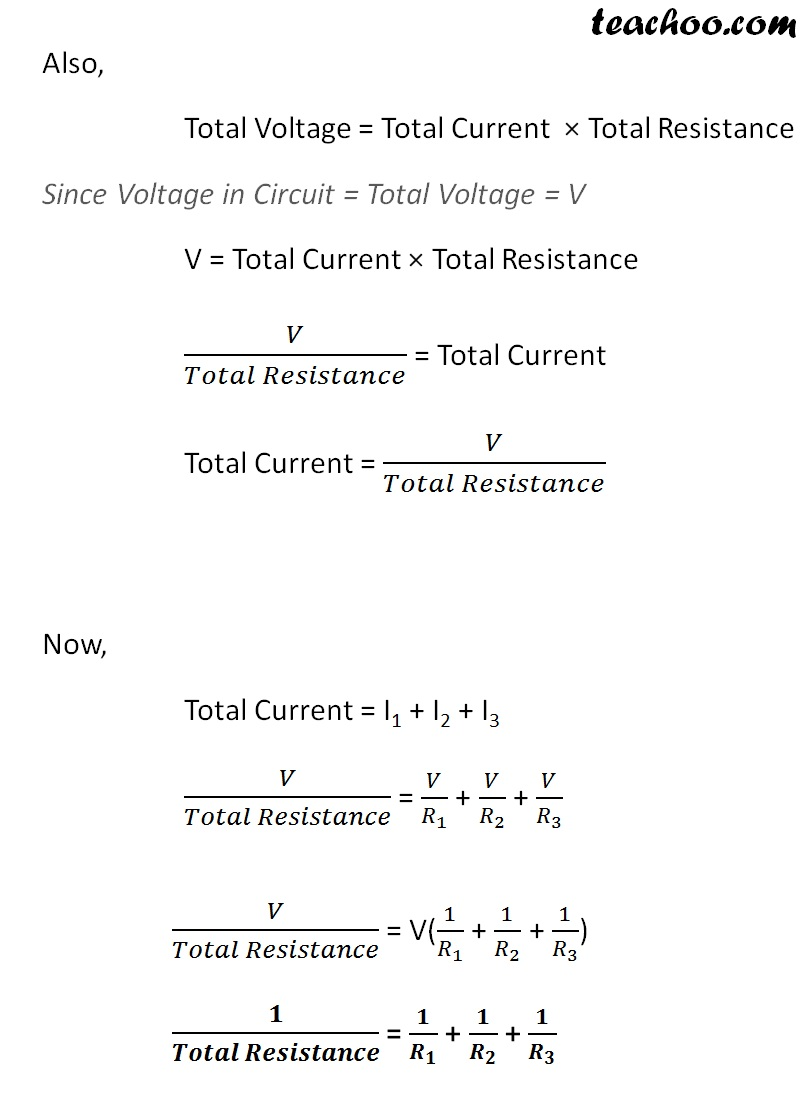Concepts

Class 10
Chapter 12 Class 10 - Electricity

Here Different Resistors are connected between 2 points parallel to each other

ExampleThis is done to decrease net Resistance of Circuit

In this case Total Resistance of circuit is equal to sum of reciprocals of Individual Resistance of the Resistors

1/R = 1/R 1 + 1/R 2 + 1/R 3IMPORTANT POINTS

## When 2 Resistors are Connected in Parallel

1. Different current flow through each resistor but overall Current flowing throughout the circuit remains the Same
Hence we can say that
Total Current = Current flowing through first Resistor + Current flowing through Second Resistor + Current flowing through Third Resistor
I = I 1 + I 2 + I 32. Potential Difference across all  both Resistors remain the same (as they are both between Same points)
Hence we can say that
Potential Diff=V
It is same for both Resistor 1 and Resistor 2Now we know that as per Ohm's Law

Potential Diff=Current*Resistance

Potential Difference/Resistance=Current

Current=Potential Diff/Resistance

I = V/R

## How is the Resistance formula Derived - for parallel circuits?Note - In case of parallel, the total resistance is always less than the resistance of the individual resistors.

The net resistance produced is lowest when resistors are connected in parallel.

## Questions

NCERT Question 5 - How is a voltmeter connected in the circuit to measure the potential difference between two points?

Q1 Page 216 - Judge the equivalent resistance when the following are connected in parallel –
(a) 1 Ω and 10 6 Ω,
(b) 1 Ω and 10 3 Ω, and 10 6 Ω.

Q2 Page 216 - An electric lamp of 100 Ω, a toaster of resistance 50 Ω, and a water filter of resistance 500 Ω are connected in parallel to a 220 V source. What is the resistance of an electric iron connected to the same source that takes as much current as all three appliances, and what is the current through it?

Q4 Page 216 - How can three resistors of resistances 2 Ω, 3 Ω, and 6 Ω be connected to give a total resistance of (a) 4 Ω, (b) 1 Ω?

Q5 Page 216 - What is (a) the highest, (b) the lowest total resistance that can be secured by combinations of four coils of resistance 4 Ω, 8 Ω, 12 Ω, 24 Ω?

Example 12.8 - In the circuit diagram given in Fig. 12.10, suppose the resistors R 1 , R 2 and R 3 have the values 5 Ω, 10 Ω, 30 Ω, respectively, which have been connected to a battery of 12 V. Calculate
(a) the current through each resistor,
(b) the total current in the circuit, and
(c) the total circuit resistance.

Example 12.9 - If in Fig. 12.12, R 1 = 10 Ω, R 2 = 40 Ω, R 3 = 30 Ω, R 4 = 20 Ω, R 5 = 60 Ω, and a 12 V battery is connected to the arrangement. Calculate
(a) the total resistance in the circuit, and
(b) the total current flowing in the circuit.

Question 5 - What is the minimum resistance which can be made using five resistors each of 1/5 Ω?

(a) 1/5 Ω

(b) 1/25 Ω

(c) 1/10 Ω

(d) 25 Ω

Learn in your speed, with individual attention - Teachoo Maths 1-on-1 Class

### Transcript

Example If 2 Resistors - 3 Ω and 4 Ω are connected in parallel. What is the resistance of the circuitSince resistors are connected in parallel, Resistance of Circuit is given by 1/𝑅 = 1/𝑅_1 + 1/𝑅_2 1/𝑅 = 1/3 + 1/4 1/𝑅 = (4 + 3)/(3 × 4) 1/𝑅 = 7/12 R = 12/7 R = 1.71 Ω How is the Resistance formula Derived - for Parallel circuits? In parallel circuit, Voltage is same Let Voltage in circuit = V Now, Total Current = Current in R1 + Current in R2 + Current in R3 Current in R1 V = I1 × R1 𝑉/𝑅_1 = I1 I1 = 𝑉/𝑅_1 Current in R2 V = I2 × R2 𝑉/𝑅_2 = I2 I2 = 𝑉/𝑅_2 Current in R3 V = I3 × R3 𝑉/𝑅_3 = I3 I3 = 𝑉/𝑅_3 Also, Total Voltage = Total Current × Total Resistance Since Voltage in Circuit = Total Voltage = V V = Total Current × Total Resistance 𝑉/(𝑇𝑜𝑡𝑎𝑙 𝑅𝑒𝑠𝑖𝑠𝑡𝑎𝑛𝑐𝑒) = Total Current Total Current = 𝑉/(𝑇𝑜𝑡𝑎𝑙 𝑅𝑒𝑠𝑖𝑠𝑡𝑎𝑛𝑐𝑒) Now, Total Current = I1 + I2 + I3 𝑉/(𝑇𝑜𝑡𝑎𝑙 𝑅𝑒𝑠𝑖𝑠𝑡𝑎𝑛𝑐𝑒) = 𝑉/𝑅_1 + 𝑉/𝑅_2 + 𝑉/𝑅_3 𝑉/(𝑇𝑜𝑡𝑎𝑙 𝑅𝑒𝑠𝑖𝑠𝑡𝑎𝑛𝑐𝑒) = V(1/𝑅_1 + 1/𝑅_2 + 1/𝑅_3 ) 𝟏/(𝑻𝒐𝒕𝒂𝒍 𝑹𝒆𝒔𝒊𝒔𝒕𝒂𝒏𝒄𝒆) = 𝟏/𝑹_𝟏 + 𝟏/𝑹_𝟐 + 𝟏/𝑹_𝟑